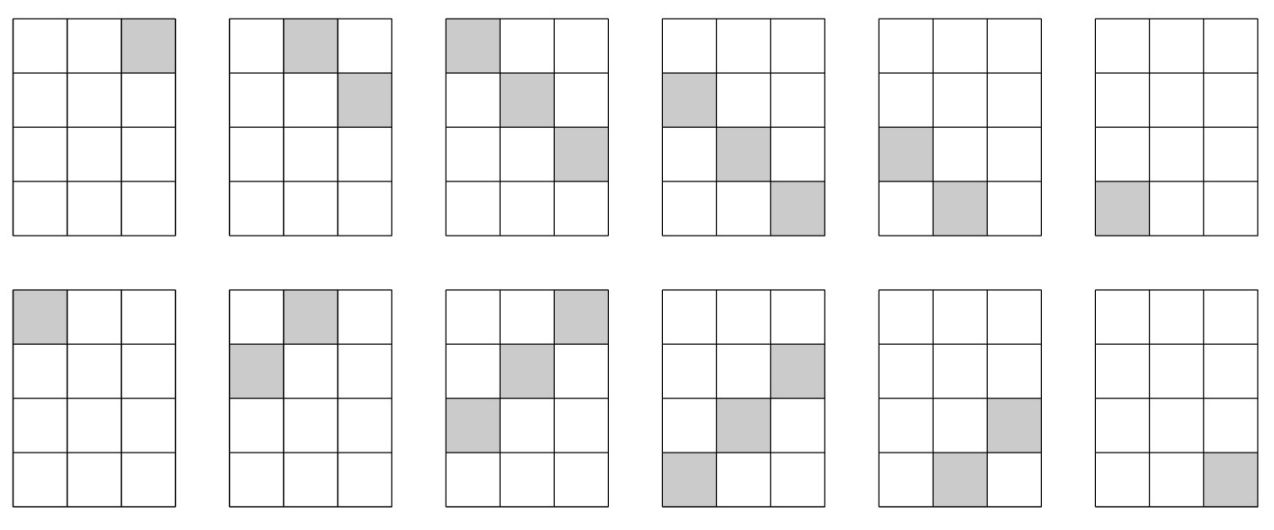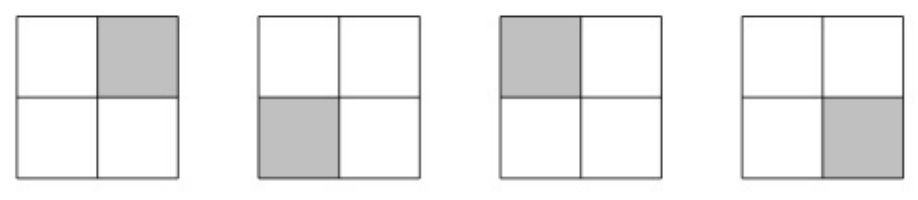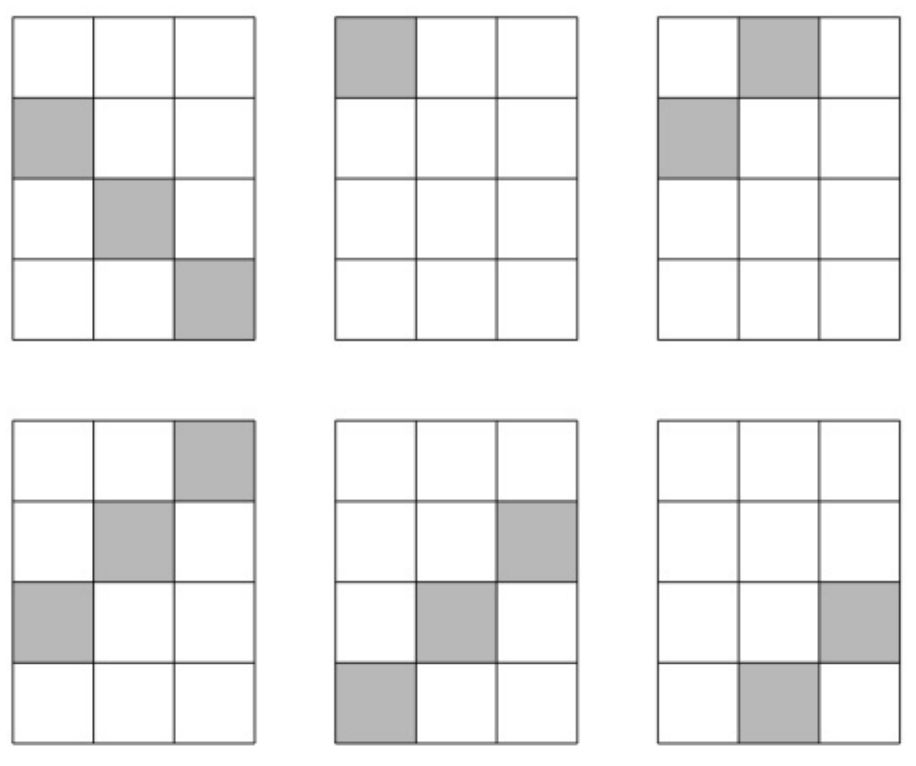시간 제한 메모리 제한 제출 정답 맞은 사람 정답 비율
2 초 512 MB 0 0 0 0.000%

## 문제

Srečko would like to paint a rectangular grid having m rows (numbered from 0 to m-1) and n columns (numbered from 0 to n-1). Initially, all cells in the grid are white. In each step, he chooses a diagonal and paints all of its cells using his favourite colour. However, some diagonals may be more expensive to paint than others, regardless of their length. Given the cost of painting each of the diagonals, write a program to tell Srečko the minimum total cost of painting all cells in the grid. Note that cells can be painted twice.

A rectangular grid with m rows and n columns has 2m + 2n - 2diagonals. For instance, if m = 4 and n = 3, there are 12 diagonals:## 입력

The input consists of three lines.

The first line contains the numbers m and n.

The second line contains m + n - 1 numbers that specify the costs of painting the diagonals running in the ↘ direction. The i-th number (for i ∈ {1, ..., m + n - 1) refers to the diagonal in which the difference between the row index and the column index is i - n. The first number thus refers to the diagonal that consists only of the cell (0, n-1) (row 0, column n-1), the second number refers to the diagonal comprising the cells (0, n-2) and (1, n-1), etc. The order of the diagonals is thus the same as in the first row of the above figure.

The third line contains m + n - 1 numbers that specify the costs of painting the diagonals running in the ↗ direction. The i-th number (for i ∈ {1, ..., m + n - 1}) refers to the diagonal in which the sum of the row index and the column index is i - 1. The first number thus refers to the diagonal that consists only of the cell (0, 0), the second number refers to the diagonal comprising the cells (1, 0) and (0, 1), etc. The order of the diagonals is thus the same as in the second row of the above figure.

## 출력

Output the minimum cost of painting the grid.

## 제한

• 1 ≤ m ≤ 2·105
• 1 ≤ n ≤ 2·105
• The costs are integers from the interval [1,109]

## 예제 입력 1

2 2
1 3 1
1 3 1


## 예제 출력 1

4


In this case, the following diagonals have to be painted to minimize the total cost:Each of the selected diagonals costs 1, so the total cost is 4.

## 예제 입력 2

4 3
2 3 9 3 4 3
2 3 3 1 2 4


## 예제 출력 2

14


This time, the following diagonals cover the grid at the minimum total cost:The costs of painting the selected diagonals are 3, 2, 3, 3, 1, and 2 (in this order).